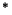Next: Examples Up: Common Syntax of the Previous: Problems

# The Logical Parts

Any non-predefined signature symbol used in a problem has to be defined in a the declaration part. Then the logical part may provide a formulation of the problem by formulae as well as by some clause normal forms. In addition, proofs for the conjecture stated by the formulae (clauses) may be contained.

 logical_part ::= {symbol_list} {declaration_list} {formula_list}{clause_list}{proof_list}As mentioned before, non-predefined signature symbols have to be declared in advance. Since the current scope of the syntax mainly covers first-order logic, we are concerned with function and predicate symbols and non-standard operators and quantifiers. The usual first-order operators and quantifiers are predefined. In addition, there is a unique symbol for equality, see below.

 symbol_list ::= list_of_symbols. {functions[fun_sym | (fun_sym,arity) {, fun_sym | (fun_sym,arity)}].} {predicates[pred_sym | (pred_sym,arity) {, pred_sym | (pred_sym,arity)}].} {sorts[sort_sym {,sort_sym}].} {operators[ op_sym | (op_sym,arity) {, op_sym | (op_sym,arity)}].} {quantifiers[quant_sym | (quant_sym,arity) {, quant_sym | (quant_sym,arity)}].} end_of_list.

All declared symbols have to be different from each other and from all terminal and predefined symbols.

We support a rich sort language that may be introduced by a declaration part. We do not allow free variables in term declarations, but polymorphic sorts.

 declaration_list ::= list_of_declarations. {declaration}end_of_list. declaration ::= subsort_decl | term_decl | pred_decl | gen_decl gen_decl ::= sort sort_sym {freely} generated by func_list. func_list ::= [ fun_sym {,fun_sym}] subsort_decl ::= subsort(sort_sym,sort_sym). term_decl ::= forall(term_list,term). | term. pred_decl ::= predicate(pred_sym{,sort_sym}+). sort_sym ::= identifier pred_sym ::= identifier fun_sym ::= identifier

Concerning the term declarations, we assume that all terms in term_list are variables or expressions of the form sort_sym(variable).

Now there are two types of formulae: Axiom formulae and conjecture formulae. If the status of the problem (see below) states ``unsatisfiable'' it refers to the clause normal form resulting from the conjunction of all axiom formulae and the negation of the conjunction of all conjecture formulae. Of course, ``satisfiable'' means that the overall formula has a model.

 formula_list ::= list_of_formulae(origin_type). {formula({term}{,label}).}end_of_list. origin_type ::= axioms | conjectures label ::= identifier

We assume that all formulae are closed, so we do not allow free variables inside a formula expression.

Quantifiers always have two arguments: A term list and the subformulae. The term list is assumed to be a variable list (or a list of variables annotated with a sort) for the usual first-order quantifiers, however, one could easily imagine non-classical quantifiers, where ``quantification'' over real terms makes sense.

 term ::= quant_sym(term_list,term) | symbol | symbol(term{,term}) term_list ::= [term{,term}] quant_sym ::= forall | exists | identifier symbol ::= equal | true | false | or | and | not | implies | implied | equiv | identifier

We support disjunctive normal form as well as clause normal form. Even clauses have to be written as their corresponding formulae, in particular all variables have to be bound by the leading quantifier. Our experience with problems stated by a set of clauses shows that this helps to detect flaws, e.g., if accidentally it was forgotten to declare some constant that would then be considered as a variable. Since free variables are not allowed, this case is detected in our syntax.

 clause_list ::= list_of_clauses(origin_type,clause_type). {clause({cnf_clause | dnf_clause}{,label}).}end_of_list. clause_type ::= cnf | dnf cnf_clause ::= forall(term_list,cnf_clause_body) | cnf_clause_body dnf_clause ::= exists(term_list,dnf_clause_body) | dnf_clause_body cnf_clause_body ::= or(term{,term}) dnf_clause_body ::= and(term{,term})

In case of cnf_clause_body and dnf_clause_body we assume all subterms generated for term to be literals.

The alphabet allowed to compose identifiers is restricted to letters, digits and the underscore symbol.

 identifier ::= {letter | digit | special_symbol}+ letter ::= a -z | A -Z arity ::= -1 | number number ::= {digit}+ digit ::= 0 | 1 | 2 | 3 | 4 | 5 | 6 | 7 | 8 | 9 special_symbol ::= _Next: Examples Up: Common Syntax of the Previous: Problems
Uwe Brahm
1999-09-15